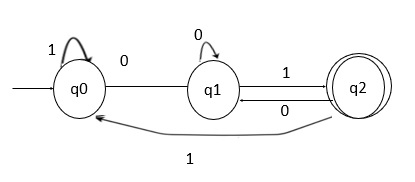# C Program to build DFA accepting the languages ending with “01”

## Problem

Design deterministic finite automata (DFA) with ∑ = {0, 1} that accepts the languages ending with “01” over the characters {0, 1}.

## Solution

The strings that are generated for a given language are as follows −

L={01,001,101,110001,1001,……….}

The minimum length of the string is 2, the number of states that the DFA consists of for the given language is: 2+1 = 3 states.Here,

• q0 − On input 0 it goes to state q1 and on input 1 it goes to itself.

• q1 − On input 0 it goes to itself and on input 1 it goes to State q2.

• q2 − On input 0 it goes to State q1 and on input 1 goes to State q0. State to q2 is the final state.

## Example

Following is the C program to construct a DFA with ∑ = {0, 1} that accepts the languages ending with “01” over the characters {0, 1} -

Live Demo

#include
#define max 100
main() {
char str[max],f='a';
int i;
printf("enter the string to be checked: ");
scanf("%s",str);
for(i=0;str[i]!='\0';i++) {
switch(f) {
case 'a': if(str[i]=='0') f='b';
else if(str[i]=='1') f='a';
break;
case 'b': if(str[i]=='0') f='b';
else if(str[i]=='1') f='c';
break;
case 'c': if(str[i]=='0') f='b';
else if(str[i]=='1') f='a';
break;
}
}
if(f=='c')
printf("String is accepted", f);
else printf("String is not accepted", f);
return 0;
}

## Output

The output is as follows −

Run 1:
enter the string to be checked: 10101001
String is accepted.
Run 2:
enter the string to be checked: 10000010
String is not accepted.